# Knowledge Distillation in a Deep Neural Network

## Distilling knowledge from a Teacher to a Student in a Deep Neural Network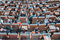Photo by Mikael Kristenson on Unsplash

In this article, you will learn.

• An easy to understand explanation of Teacher-Student knowledge distillation neural networks
• Benefits of Knowledge distillation
• Implementation of Knowledge distillation on the CIFAR-10 dataset

Large deep neural networks or an ensemble of deep neural networks built for better accuracy are computationally expensive, resulting in longer inference times which may not be ideal for near-real-time inference required at the Edge.

The training requirements for a model are different from requirements at the inference. During training, an Object recognition model must extract structure from very large, highly redundant datasets but, during inference, must operate in real-time with stringent requirements on latency and computational resources.

To address the latency, accuracy, and computational needs at the inference time, we can use model compression techniques like

• Model Quantization, low-precision arithmetic for inference, like converting a float to an unsigned int.
• Pruning, removing weights or activations that are close to zero resulting in a smaller model
• Knowledge Distillation where a large complex model(teacher) distills its knowledge and passes it to train a smaller network(student) to match the output. The student network is trained to match the larger network's prediction and the distribution of the teacher's network.

Knowledge Distillation is a model-agnostic technique to compresses and transfers the knowledge from a computationally expensive large deep neural network(Teacher) to a single smaller neural work(Student) with better inference efficiency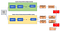Image by author

Knowledge Distillation consists of two neural networks: Teacher and Student models.

1. Teacher Model: A larger cumbersome model can be an ensemble of separately trained models or a single very large model trained with a very strong regularizer such as dropout. The cumbersome model or the Teacher model is trained first.
2. Student Model: A smaller model which will use the distilled knowledge from the Teacher network. It uses a different kind of training, referred to as “distillation,” to transfer the knowledge learned from the cumbersome model to a smaller Student model. The student model is more suitable for deployment as it will be computationally inexpensive with the same or better accuracy than the Teacher model.

Knoweldeg distillation extracts the knowledge from the large cumbersome Teacher model and passes it on to the smaller Student model.

How is knowledge distilled from the Teacher to the Student model?

Knowledge is distilled from large, complex Teacher model to Student model using Distillation loss.

Knowledge distillation uses generalization using soft targets that mitigate the over-confidence issue of neural networks and improves model calibration.

Distillation loss uses the soft targets to minimize the squared difference between the logits produced by the cumbersome model and the logits produced by the small model.

In knowledge distillation, knowledge is transferred to the distilled model by training the cumbersome model with a high temperature in its softmax to generate soft target distribution. The same high temperature is used for training the distilled model, but after it has been trained, it uses a temperature of 1.

Knowledge distillation minimizes the KL divergence between a teacher and student network's probabilistic outputs in the distilled model. KL divergence constraints the student model's outputs to match soft targets of the large, cumbersome model.

What are Soft targets and Hard targets?

Hard targets are generated when using a softmax function. Using the softmax function, the model almost always produces the correct answer with very high confidence, which has very little influence on the cross-entropy cost function during the transfer of knowledge from Teacher to Student because the probabilities are so close to zero.

Soft targets use the logits, the inputs to the final softmax rather than the softmax's probabilities as the targets for learning the small model.

When the soft targets have high entropy, they provide much more information per training case than hard targets. They also have less variance in the gradient between training cases.

Hard Targets are generated using the Softmax activation function that converts the logit, zi, computed for each class into a probability,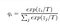Soft targets

Soft targets are computed as qi, by comparing zi with the other logits where T is a temperature.

When T=1, it is the same as the softmax activation function. A higher value for T produces a softer probability distribution over classes, as shown below.

`import numpy as nplogits= np.array([0,1,0,0,0])T=[1,5,7,10]for t in T:    logits_exp_norm= np.exp(logits)/sum(np.exp(logits))    logits_exp_norm_with_T= np.exp(logits/t)/sum(np.exp(logits/t))    print("with T=",t, logits_exp_norm_with_T)print("softmax :", logits_exp_norm)`
• Soft targets contain valuable information on the rich similarity structure over the data, i. e. it says which 2 looks like 3’s and which looks like 7’s.
• Provides better generalization and less variance in gradients between training examples.
• Allows the smaller Student model to be trained on much smaller data than the original cumbersome model and with a much higher learning rate
• Can be deployed at the Edge: Knowledge distillation, a student model, inherits better quality from the teacher and is more efficient for inference due to its compactness needing less computational resources.
• Improves generalization: The teacher produces “soft targets” for training the student model. Soft targets have high entropy providing more information than one hot encoded hard target. Soft targets have less variance in the gradient between training cases allowing student networks to be trained on much less data than the Teacher using a much higher learning rate.

Import required libraries

`import tensorflow as tfimport numpy as np`

Creating the CIFAR-10 train and test dataset

`# Prepare the train and test dataset.batch_size = 64(x_train, y_train), (x_test, y_test) = tf.keras.datasets.cifar10.load_data()# Normalize datax_train = x_train.astype("float32") / 255.0x_train = np.reshape(x_train, (-1, 32, 32, 3))x_test = x_test.astype("float32") / 255.0x_test = np.reshape(x_test, (-1, 32, 32,3))print("Input Train data  ",x_train.shape)print("Train data Labels ",y_train.shape)print("Input Test data   ",x_test.shape)print("Test data Labels  ",y_test.shape)`

Create the Cumbersome Teacher model

`teacher = tf.keras.Sequential(    [        tf.keras.Input(shape=(32, 32, 3)),                tf.keras.layers.Conv2D(256, (3, 3), strides=(2, 2), padding="same"),        tf.keras.layers.LeakyReLU(alpha=0.2),        tf.keras.layers.MaxPooling2D(pool_size=(2, 2), strides=(1, 1), padding="same"),        tf.keras.layers.Conv2D(512, (3, 3), strides=(2, 2), padding="same"),        tf.keras.layers.LeakyReLU(alpha=0.2),        tf.keras.layers.MaxPooling2D(pool_size=(2, 2), strides=(1, 1), padding="same"),        tf.keras.layers.Flatten(),        tf.keras.layers.Dense(10),    ],    name="teacher",)teacher.summary()`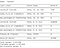Cumbersome Teacher Model

Create the simple Student model

`# Create the studentstudent = tf.keras.Sequential(    [        tf.keras.Input(shape=(32, 32, 3)),        tf.keras.layers.Conv2D(64, (3, 3), strides=(2, 2), padding="same"),        tf.keras.layers.LeakyReLU(alpha=0.2),        tf.keras.layers.MaxPooling2D(pool_size=(2, 2), strides=(1, 1), padding="same"),        tf.keras.layers.Conv2D(256, (3, 3), strides=(2, 2), padding="same"),        tf.keras.layers.LeakyReLU(alpha=0.2),        tf.keras.layers.MaxPooling2D(pool_size=(2, 2), strides=(1, 1), padding="same"),        tf.keras.layers.Flatten(),        tf.keras.layers.Dense(10),    ],    name="student",)student.summary()`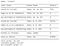Simple Student Model

You can see that our Teacher Model has approximately 1.51M parameters and will be computationally very expensive when compares to the smaller, simpler Student Model with just 313K parameters.

Train the Teacher Model

`teacher.compile(    optimizer=tf.keras.optimizers.Adam(),    loss=tf.keras.losses.SparseCategoricalCrossentropy(from_logits=True),metrics=[tf.keras.metrics.SparseCategoricalAccuracy()],)# Train and evaluate teacher on data.teacher.fit(x_train, y_train, epochs=5)teacher.evaluate(x_test, y_test)`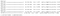Teacher model Training and Evaluation

Distill the Knowledge from the Teacher Model to the Student Model

Create a Distiller class to distill the knowledge using the student and distillation loss.

• The student loss function is the difference between student predictions and ground-truth using the softmax function where T=1.
• The distillation loss function is the difference between the soft student predictions and the soft teacher labels.

Code Adapted and Inspired by: //keras.io/examples/vision/knowledge_distillation/

`class Distiller(keras.Model):    def __init__(self, student, teacher):        super(Distiller, self).__init__()        self.teacher = teacher        self.student = studentdef compile(        self,        optimizer,        metrics,        student_loss_fn,        distillation_loss_fn,        alpha=0.1,        temperature=3,    ):        """ Configure the distiller.student_loss_fn: Loss function of difference between student                predictions and ground-truthdistillation_loss_fn: Loss function of difference between soft                student predictions and soft teacher predictionsalpha: weight to student_loss_fn and 1-alpha to distillation_loss_fntemperature: Temperature for softening probability distributions.                Larger temperature gives softer distributions.        """        super(Distiller, self).compile(optimizer=optimizer, metrics=metrics)        self.student_loss_fn=student_loss_fn        self.distillation_loss_fn= distillation_loss_fn        self.temperature= temperature        self.alpha= alpha            def train_step(self, data):        x,y=data                # Forward pass of teacher        teacher_prediction=self.teacher(x, training=False)        print("Tecaher prediction   ...", teacher_prediction)        with tf.GradientTape() as tape:            # Forward pass of student            student_predcition= self.student(x, training=True)            # Compute losses            student_loss= self.student_loss_fn(y, student_predcition)                        distillation_loss=self.distillation_loss_fn(            tf.nn.softmax(teacher_prediction/self.temperature, axis=1),            tf.nn.softmax(student_predcition/self.temperature, axis=1)            )            loss= self.alpha* student_loss + (1-self.alpha)* distillation_loss            print("Loss in distiller :",loss)            # Compute gradients            trainable_vars= self.student.trainable_variables            gradients=tape.gradient(loss, trainable_vars)            gradients = [gradient * (self.temperature ** 2) for gradient in gradients]            # Update weights            self.optimizer.apply_gradients(zip(gradients, trainable_vars))                        # Update the metrics configured in `compile()`            self.compiled_metrics.update_state(y, student_predcition)                        # Return a dict of performance            results={ m.name: m.result()  for m in self.metrics}            results.update({"student_loss": student_loss, "distillation_loss": distillation_loss})            print("Train...", results)            return results            def test_step(self, data):        # Unpack the data        x, y = data                ## Compute predictions        y_prediction= self.student(x, training=False)                # calculate the loss        student_loss= self.student_loss_fn(y, y_prediction)                # Update the metrics.        self.compiled_metrics.update_state(y, y_prediction)                # Return a dict of performance        results ={m.name: m.result() for m in self.metrics}        results.update({"student_loss": student_loss})        print("Test...", results)        return results# Initialize  distillerdistiller= Distiller(student=student, teacher=teacher)`

In the above code, we override the compile, train_step, and test_step of the Model class.

We perform a forward pass on both the Teacher and Student model during training and calculate the loss as shown below, and then perform a backward pass.

where alpha is a factor to weigh the student and distillation loss.

calculate the gradients for the student weights as only the student weights are updated

During the test step, we evaluate the student model using the student prediction and the ground truth.

Compiling the distiller

`#compile distillerdistiller.compile(optimizer=keras.optimizers.Adam(),                 metrics=[keras.metrics.SparseCategoricalAccuracy()],                 student_loss_fn=keras.losses.SparseCategoricalCrossentropy(from_logits=True),                 distillation_loss_fn=keras.losses.KLDivergence(),                 alpha=0.3,                 temperature=7)`

The Distillation model uses distillation loss minimized using the KL divergence between the probabilistic outputs of a teacher and student network in the distilled model.

Distill the knowledge from the Teacher model to Student and Evaluate the distiller

`# Distill teacher to studentdistiller.fit(x_train, y_train, epochs=5)# Evaluate student on test datasetdistiller.evaluate(x_test, y_test)`

We can see that the Teacher model, on average, tasks 12 sec per epoch to train, whereas the distiller takes 9sec on average per epoch to train.

The Accuracy of the Teacher model with 5 epochs is 68.8%, whereas the accuracy of the Distillation Model 71.1%

Conclusion:

Knowledge distillation is a model agnostic compression technique that extracts the knowledge from the large cumbersome Teacher model and passes it on to the smaller Student model. The Knowledge distillation model uses soft targets and has less training and inference time but a higher accuracy than the large cumbersome Teacher Model.

Distilling the Knowledge in a Neural Network: Geoffrey Hinton, Oriol Vinyal, and Jeff Dean

https://keras.io/examples/vision/knowledge_distillation/

https://www.youtube.com/watch?v=k63qGsH1jLo&t=258s

## Analytics Vidhya

Analytics Vidhya is a community of Analytics and Data…

## Sign up for Analytics Vidhya News Bytes

### By Analytics Vidhya

Latest news from Analytics Vidhya on our Hackathons and some of our best articles! Take a look.

By signing up, you will create a Medium account if you don’t already have one. Review our Privacy Policy for more information about our privacy practices.

Check your inbox
Medium sent you an email at to complete your subscription.

Written by

## Renu Khandelwal

Loves learning, sharing, and discovering myself. Passionate about Machine Learning and Deep Learning

## Analytics Vidhya

Analytics Vidhya is a community of Analytics and Data Science professionals. We are building the next-gen data science ecosystem https://www.analyticsvidhya.com

Written by

## Renu Khandelwal

Loves learning, sharing, and discovering myself. Passionate about Machine Learning and Deep Learning## Analytics Vidhya

Analytics Vidhya is a community of Analytics and Data Science professionals. We are building the next-gen data science ecosystem https://www.analyticsvidhya.com

## More From Medium

Medium is an open platform where 170 million readers come to find insightful and dynamic thinking. Here, expert and undiscovered voices alike dive into the heart of any topic and bring new ideas to the surface. Learn more

Follow the writers, publications, and topics that matter to you, and you’ll see them on your homepage and in your inbox. Explore

If you have a story to tell, knowledge to share, or a perspective to offer — welcome home. It’s easy and free to post your thinking on any topic. Write on Medium